Identifying Functions
Describing Functions
Let statements
Equations
Word Problems
100

Is this relationship a function?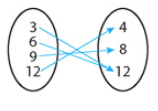yes, this is a function because every input has one output.

100

What is the rate of change?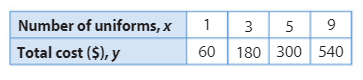60

100

Define the x and y variables.let x = the number of hours

let y = the cost

100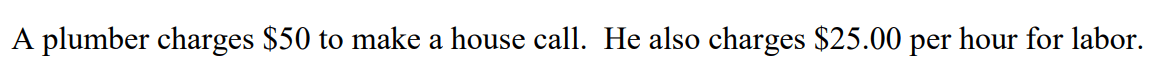y=25x+50

100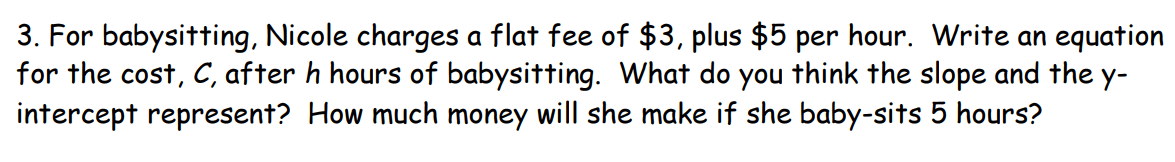Nicole will earn $28 babysitting. 200 Is this relationship a function?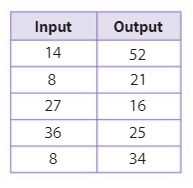No, this is not a function because an input of 8 has outputs of 21 and 34. 200 What is the slope and y-intercept?m=-1/2 and b=1 200 Define the x and y variables.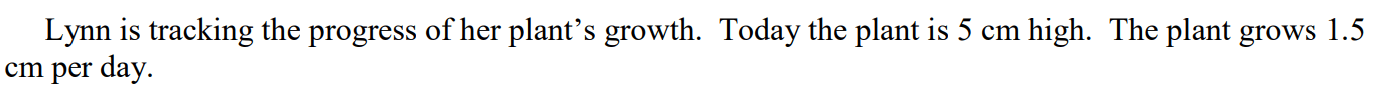let x = the number of days let y = the height of the plant 200y=1.5x+5 200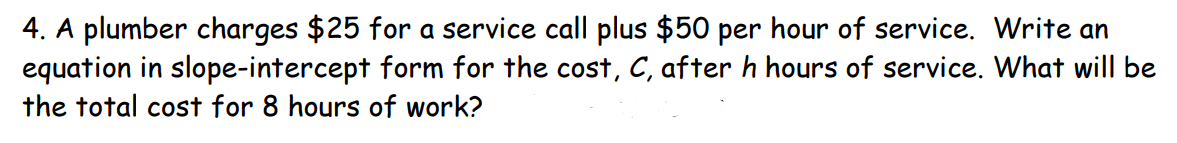The plumber will earn$425.

300

Is this relationship a function?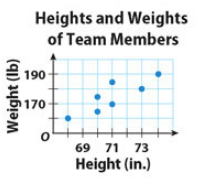No, this is not a function because an input of 70 has two outputs.

300

What is the slope and y-intercept?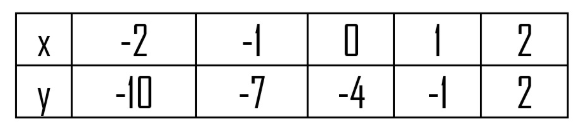m=3 and b=-4

300

Define the x and y variables.let x = the number of seconds

let y = the plane's altitude

300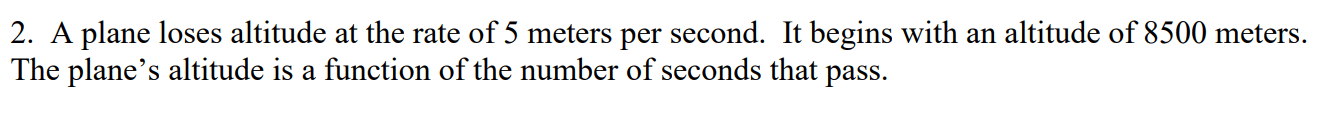y=-5x+8500

300There is 255 gallons of water in the tank.

400

Is this relationship a function?yes, this is a function because every input has one output.

400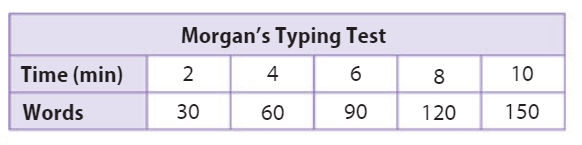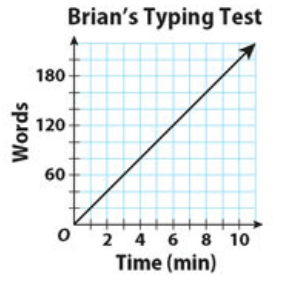Morgan types 15 words per minute.

Brian types 20 words per minute.

Brian types faster than Morgan.

400

Define the x and y variables.let x = the number of months

let y = the cost of internet service

400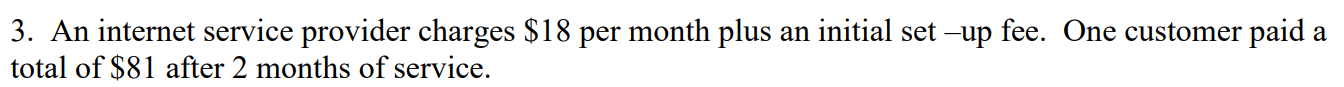y=18x+45

400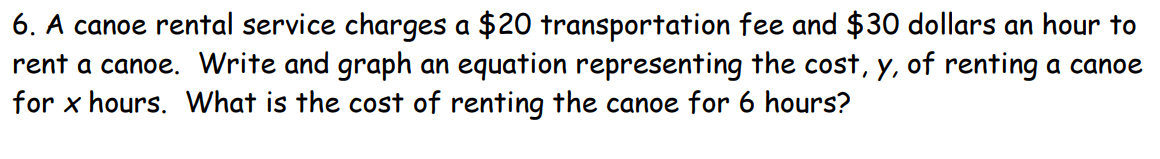It costs $200 to rent the canoe for 6 hours. 500 Is this relationship a function?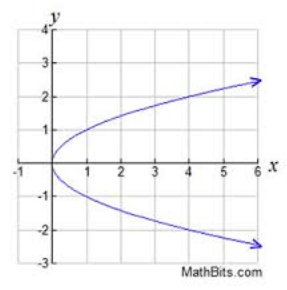No, this is not a function because an input of 4 has outputs of 2 and -2. 500 Which plan is cheaper if a student needs to be tutored for 6 hours?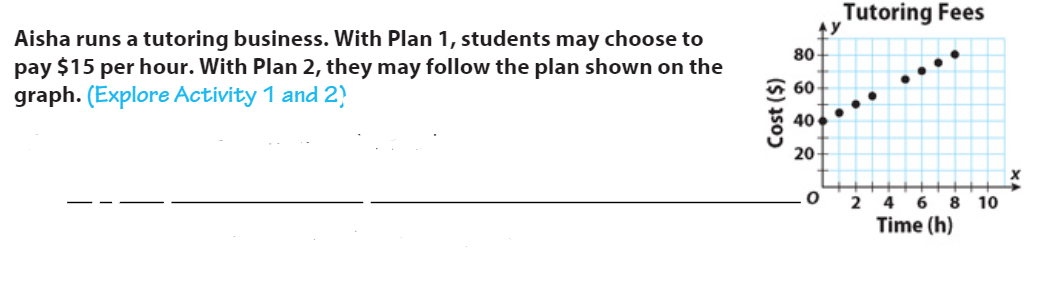Plan 2 500 Define the x and y variables.let x = the number of months let y = the cost of the gym membership 500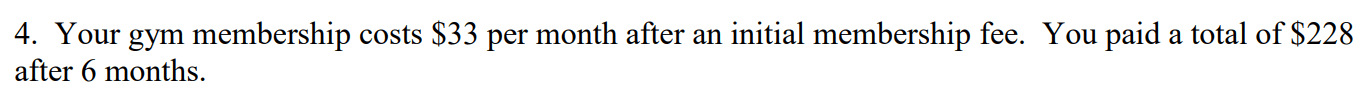y=33x+30 500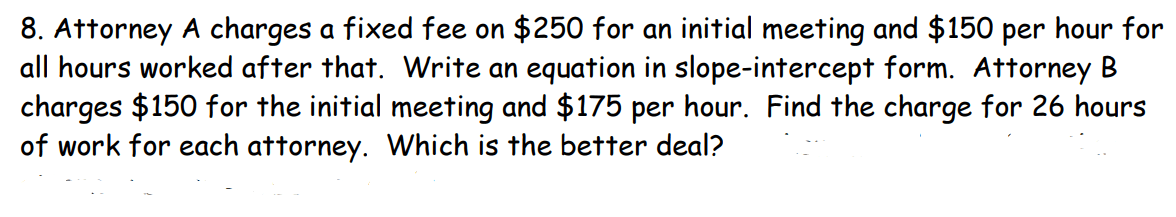Attorney A charges$4,150 for 26 hours.

Attorney B charges \$4,700 for 26 hours.

Attorney A has the better price.

Click to zoom